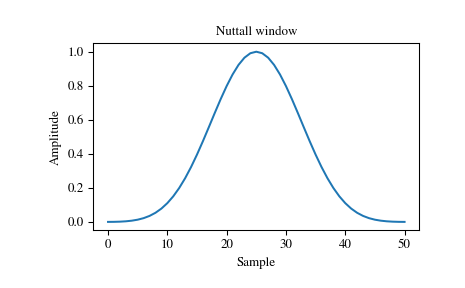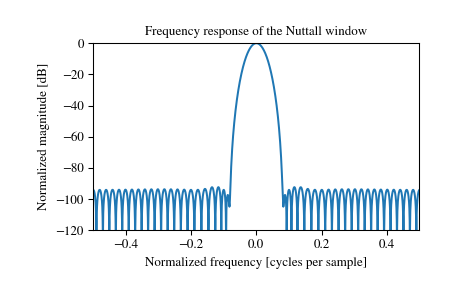# scipy.signal.windows.nuttall¶

scipy.signal.windows.nuttall(M, sym=True)[source]

Return a minimum 4-term Blackman-Harris window according to Nuttall.

This variation is called “Nuttall4c” by Heinzel. 

Parameters: M : int Number of points in the output window. If zero or less, an empty array is returned. sym : bool, optional When True (default), generates a symmetric window, for use in filter design. When False, generates a periodic window, for use in spectral analysis. w : ndarray The window, with the maximum value normalized to 1 (though the value 1 does not appear if M is even and sym is True).

References

  A. Nuttall, “Some windows with very good sidelobe behavior,” IEEE Transactions on Acoustics, Speech, and Signal Processing, vol. 29, no. 1, pp. 84-91, Feb 1981. DOI:10.1109/TASSP.1981.1163506.
  (1, 2) Heinzel G. et al., “Spectrum and spectral density estimation by the Discrete Fourier transform (DFT), including a comprehensive list of window functions and some new flat-top windows”, February 15, 2002 https://holometer.fnal.gov/GH_FFT.pdf

Examples

Plot the window and its frequency response:

>>> from scipy import signal
>>> from scipy.fftpack import fft, fftshift
>>> import matplotlib.pyplot as plt

>>> window = signal.nuttall(51)
>>> plt.plot(window)
>>> plt.title("Nuttall window")
>>> plt.ylabel("Amplitude")
>>> plt.xlabel("Sample")

>>> plt.figure()
>>> A = fft(window, 2048) / (len(window)/2.0)
>>> freq = np.linspace(-0.5, 0.5, len(A))
>>> response = 20 * np.log10(np.abs(fftshift(A / abs(A).max())))
>>> plt.plot(freq, response)
>>> plt.axis([-0.5, 0.5, -120, 0])
>>> plt.title("Frequency response of the Nuttall window")
>>> plt.ylabel("Normalized magnitude [dB]")
>>> plt.xlabel("Normalized frequency [cycles per sample]")#### Previous topic

scipy.signal.windows.kaiser

#### Next topic

scipy.signal.windows.parzen